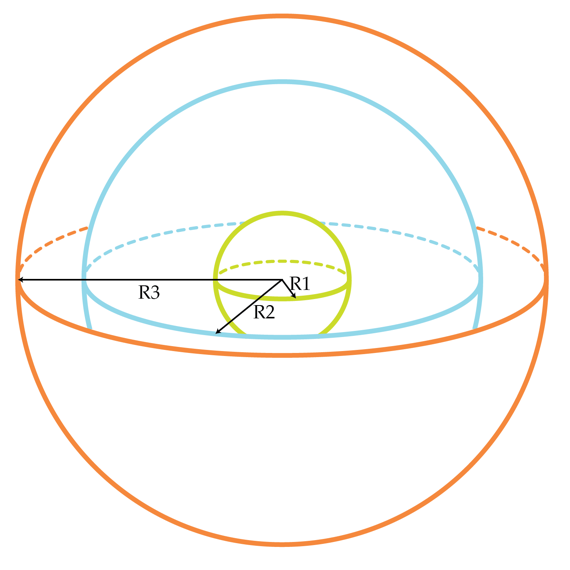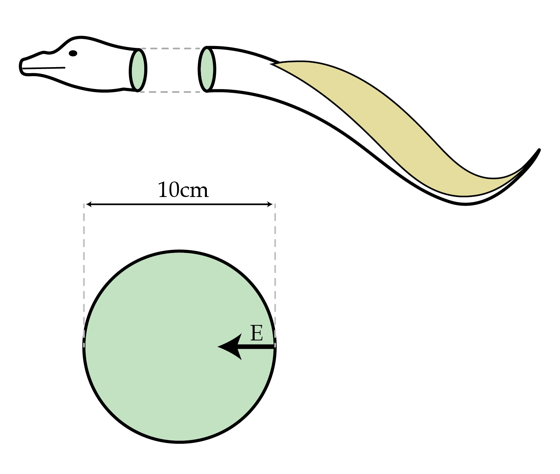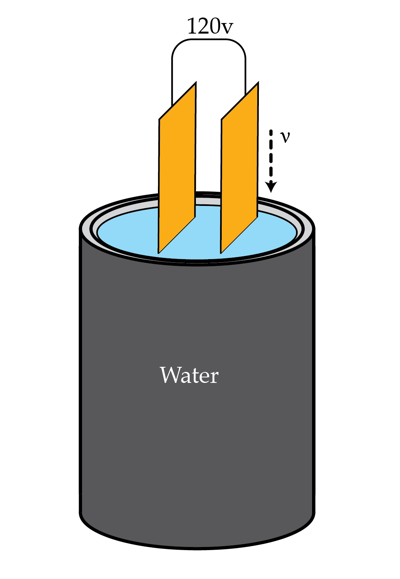Electricity and Magnetism

# Capacitors and Transformers: Level 3-4 Challenges

Consider a system of three concentric metallic spheres with radii $R_{1}= 10 cm, R_{2}=30 cm$ and $R_{3}=40 cm$. The inner ($R_{1}$) and the outer ($R_{3}$) spheres are grounded (they are connected to a large conductor at zero potential ). The relation between the charge Q of the middle sphere and its potential V is linear $Q= C_{self} V.$ The coefficient $C_{self}$ is called self-capacitance. Determine $C_{self}$ in Farads for the middle sphere.Details and assumptions

$k=\frac{1}{4\pi \epsilon_{0}}= 9\times 10^{9} m/F$

An electric eel can generate a voltage of $600~\mbox{V}$ across its body. The eel does this via a system of up to 6000 “electroplaques” inside its body. What is the average strength of the electric ﬁeld in V/m inside the body of a $10~\mbox{cm}$ wide eel?Details and assumptions

• Treat the opposite sides of the eel as the plates of a parallel plate capacitor.

A 120V source is connected across the plates of a parallel-plate capacitor. The capacitor is being submerged vertically at constant speed into a container filled with water. What current in Amps flows through the voltage source during this process if it takes $\tau=20 s$ to totally submerge the capacitor? The capacitance (in air) of the capacitor is $C_{0}=12\mu F$ and water's relative permittivity is $\epsilon=81.$Hint: If the capacitor is partially submerged at an instant of time try figuring out how to treat it as two separate capacitors at that instant.

According to the legend, Zeus, the king of ancient Greek gods, had the power to create thunderbolts in his hands and throw them at his opponents. Suppose Zeus creates the thunderbolts by bringing his palms very close together and parallel to each other and then inducing equal but opposite charge at each of them. What magnitude charge does he have to induce in nC on each palm for this to be possible?

Details and assumptions

• Zeus' hands are in air, which has approximately the same dielectric permittivity as vacuum.
• Zeus' palms can be modeled as rectangular, with the sides $20~\mbox{cm}$ and $15~\mbox{cm}$.
• The threshold for air ionization is $E = 3 \times 10^6~\mbox{V/m}$

One can make a homemade capacitor using an aluminum foil and a plastic or a wooden stick (it's important that the stick is an insulator). Make a small ball out of the aluminum foil and wrap it around the stick. Then make a wider foil sphere around the ball so that they don't touch, and your capacitor is ready!

If the radius of the smaller ball is $5~\mbox{cm}$ and of the bigger sphere $15~\mbox{cm}$, which capacitance in pF do you expect to obtain?

Details and assumptions

• The ball and the sphere are centered around the same point
×